# SPSS On-Line Training WorkshopHOME Table of Contents Data Editor Window Syntax  Editor WindowCarl Lee Felix Famoye About Us Chart Editor Window Output Window Overview of Data Analysis Manipulation of Data Analysis of Data Projects & Data Sets Integrate R into SPSS
 Data Types and Possible Analysis Techniques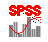We will cover:

Types of
Statistics

Descriptive &
Graphical

Inferential

Variable
Relationships

Statistical
Modeling

Data Dimension
Reduction

Nonparametric
Methods

Quality Control

Time Series

Survival Modeling

ROC Curve

Data Types

General speaking, statistical techniques are determined by the type of data. A basic understanding about the data types is helpful for choosing statistical procedures. In SPSS, a column is for a variable and a row is for a case. There are, generally speaking, two major types of data: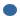Qualitative variables: The data values are non-numeric categories. Examples: Blood type, Gender.Quantitative variables: The data values are counts or numerical measurements. A quantitative variable can be either discrete such as # of students receiving an 'A' in a class, or continuous such as GPA, salary and so on.

Another way of classifying data is by the measurement scales. In statistics, there are four generally used measurement scales:Nominal data: data values are non-numeric group labels. For example, Gender variable can be defined as male = 0 and female =1.Ordinal data (we sometimes call 'Discrete Data'): data values are categorical and may be ranked in some numerically meaningful way. For example, strongly disagree to strong agree may be defined as 1 to 5.Continuous data: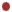Interval data : data values are ranged in a real interval, which can be as large as from negative infinity to positive infinity. The difference between two values are meaningful, however, the ratio of two interval data is not meaningful. For example temperature, IQ. Today is 1.2 times hotter than yesterday is not much useful nor meaningful.Ratio data: Both difference and ratio of two values are meaningful. For example, salary, weight.

NOTE: The statistical procedures mentioned below are demonstrated using movie clips in the Statistical Procedures Page.

In this on-line workshop, you will find many movie clips. Each movie clip will demonstrate some specific usage of SPSS.

Basic Statistics is typically divided into these areas:Descriptive statistics:Summary statistics: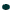meanmedianstandard deviationpercentilefrequencySummary graphic toolspie chartshistogramsboxplotsscatterplotsInferential statistics: used to make comparisons between two or more groups or study relationshipsEstimationConfidence intervalHypothesis testingStatistical Modeling: used to modeling one response variable (dependent variable) based on a list of potential predictors (independent variables, or to modeling multivariate response variables using a set of potential predictors. Common modeling techniques includeMultiple regressionLogistic regressionGeneralized linear modelsMixed modelsGeneral log-linear modelsSurvival modelsDimension reduction techniques: In many statistical applications, one often have many variables. Many of them are either not very useful or redundant for the study. It is, in many cases, important to perform data reduction by 'combining' information of a group of variables into a few smaller number of 'new' variables or by deleting statistically redundant variables prior to conducting advanced analysis. Many of these techniques are available in what has been known as 'data mining techniques. In this SPSS online training, we will only discuss some of those techniques that are considered more 'traditional' statistical techniques:Clustering analysis for grouping variables or cases.Factor analysis for reducing number of variables.Statistical Process Control techniques: These techniques are commonly used for monitoring and improving the quality of a process. Typically used techniques include:X-bar R-Charts for variable datap-, np-charts for attribute data that follows binomial rulesc- & u-charts for attribute data that follows Poisson processCapability analysis for studying the performance of a process prior and after any process improvement.Time Series Modeling: used to model the time series pattern of data. Typical techniques are ARIMA models and ARIMA models with seasonal adjustment.Survival modeling: used for data that are truncated. In this online workshop, we will talk about two survial modeling techniques:Kaplan-Meier survival modelsCox regression modelsROC Curve: A graphical technique for comparing the performances of different classification models. This technique is a model selection technique that is often used for selecting models with response variable being categorical.Design of Experimental techniques: Data are usually collected either observationally (such as survey, existing sources, etc.) or through an experiment that follows some statistical designs such as Factorial Designs, Complete Randomized Block Designs, Incomplete Block Designs, Composite Designs, Orthogonal Arrays, etc.). In this online workshop, we will not discuss techniques of experimental designs. Instead, we focus on data analysis.

Descriptive and Graphical AnalysisFor nominal data: Frequency, Crosstabs, bar charts and pie charts are common tools.For ordinal data: Frequency, Crosstabs, and descriptive statistics, bar charts, pie charts, stem-leaf plots are common tools.For continuous data: Descriptive statistics, histograms, boxplots, and scatterplots for two variables are common tools.

Inferential Analysis

If you are interested in comparing group effects.For Nominal or ordinal data: Use crosstabs.For continuous data:First, check to see if the variable is normal. To check Normality, go to 'Analyze' then to 'Descriptive Statistics' then choose 'Explore' procedure.Second, if you compare two or more groups, check the homogeneity of the variances among groups.  To do so, you also use the 'Explore' procedure.For two group comparison, use Independent t-test.For three or more group comparison, use one-way analysis of variance.For two or more factors, use multiway analysis of variance.If there are factors and covariates, use analysis of covariance.If the same subject is measured more than one time, it is a repeated measure problem.

If you are interested in the relationship between two variables:For nominal data, use crosstabs, and choose proper tests for nominal data.For ordinal data, use crosstabs, bivariate correlation such as Spearman correlation coefficient..For continuous data, use bivariate correlation such as Pearson correlation.

If you are interested in modeling a response (also called dependent variable) using predictor variables (also called independent variables):For nominal data, if the response is a binary variable (that is only two possible values such as graduate in four years or not), then, use Logistic regression model.  If the response has more than two categories, use multinomial logistic regression.For ordinal data, if the response follows Poisson distribution, use Poisson regression model.  In general, one can use log-linear models for ordinal data.In many applications, the relationship between response variable and predictors are not linear, but may be linearized. Generalized linear modeling techniques are useful.Some applications involves certain structure of relationship between response and predictor variables. Mixed models may be useful for some of these problems.Many medical data or reliability data involves with data values that are not completely observed at the end of the study (right-truncated), or some data have already evolving before the study started (left-truncated). The analysis requires special attention regarding to the information of data being 'truncated'. Survival modeling techniques are useful for modeling these types of data.Most of statistical techniques require certain assumptions. Typically, for continuous response, the assumptions may include normality of the response variable, homogeneity of variance and the relationship between Y and X's being linear or not. One should take appropriate data transformation as needed when building statistical mdodels.

If you are interested in reducing the data dimension: Cluster Analysis and   Factor Analysis.

Cluster analysis can be applied to group variables or cases. It is often called non-supervised techniques for the reason that this technique groups the variables (or cases) based on certain given similarity or non-similarity distance measures to group variables (or cases)  into a smaller number of groups. The variables ( or cases) in each group are similar in terms of the given distance criterion. They often share some common characteristics, which are investigated and identified by the researcher. The variables (or cases) in each group are often combined using certain linearly weighted technique to redefine a new 'combined' variable (or cases) for further analysis.

Be aware of the different between Cluster analysis and  classification analysis.Classification techniques, which are often called as 'supervised techniques', are techniques involve with classifying cases of a categorical response variable based on a set of independent variables (also called Input variables) by building a model. The model is then applied to classify future cases into one of the categories of the response based on the observed data values of the independent variables.While cluster analysis does not involve with the modeling. You have a set of k variables, each with n cases. The cluster analysis for cases will group the cases into small number of groups of cases based on the similarity of the independent variables. The cluster analysis for variables will group the variables into small number of subsets of variables based on the similarity of cases.

Factor analysis combines similar variables together into a dimension that can be interpreted from the qualitative aspects of the study. In many survey studies, one may collect many variables. It is difficult to understand the overall meaning of these variables. Factor analysis helps to combine similar variables into the same dimension, and results to only a few dimensions (factors) that are meaningful for explaining the problem.For example, in the technology survey, we collect 16 variables related to the difficulty faced by faculty when using classroom technology. Using Factor Analysis, we are able to combine these 16 different types of difficulties into four general groups of difficulty (factors). These are difficulties related to: (1) Computer hardware and software, (2) Media technology, (3) Instructor's technology need in office, and (4) Classroom instructional technology need.

Nonparametric Methods are another alternative:

If assumptions are violated for the statistical procedure that is chosen, there are many nonparametric statistical procedures that can do similar analysis that are less sensitive to the assumptions. The corresponding nonparametric procedures in SPSS include:Chi-squareTwo Independent Samples Comparison: The similar parametric procedure is independent t-test.K Independent Samples Comparison : The similar parametric procedure is Analysis of Variance.Two Related Samples: The similar parametric procedure is the paired t-test.K Related Samples: The similar parametric procedure is the Repeated Measure Analysis.

To perform nonparametric statistical procedure in SPSS,

Go to 'Analyze', go down to 'Nonparametric Tests', then select the appropriate nonparametric procedure.

Quality Control:

Statistical quality control techniques are commonly used in monitoring the process quality. There are typically two major sources of variations occurred in a process. One is the variation due to special causes, and the other is the variation due to system causes. Control charts are commonly used for monitoring the variation due to special causes. Capability analysis is typically used to evaluate the performance of the existing system. Once the capability of a system is assessed, one can then, design further investigation to study the possible factors (causes) that may result in the system variation.  There are various tools available in SPSS for quality control, include:Capability Analysis for evaluating the performance of the quality characteristic in interval scale.  Typical capability indices include Cp, Cpu, Cpl, Cpk, CpM.Variable control charts: X-bar/R charts and X-bar/S charts for monitoring variable data (interval data). X-bar chart monitors the average performance of the quality characteristic along the time. The range/s-charts monitor the variability of the quality catachrestic along the time. The assumption is the quality characteristic follows a normal distribution. Caution should be taken for situations where the normality assumption is highly violated.Variable control charts: Individual, Moving Range charts for monitoring variable (interval) measurement where each sample is taken only from one individual unit. The point represents the moving average or moving range of at least two consecutive individual measurements. Typical assumption is the number of defectives in a random sample of n items follows a binomial distributionAttribute control charts: p-, np-charts for monitoring proportion (p-chart) or number ( np-chart) of defectives of the quality characteristic in each batch of a random sample along the time.Attribute control charts: c, u-charts for monitoring the number of defects in the sample (c-chart) or the mean number of defects in one unit of sample (u-chart). The typical assumption is the number of defects in a sample follows a Poisson distribution.

To performance Quality Control procedure in SPSS,

Go to Analyze menu, select Quality Control , and click on Control Charts procedure.

Time Series:

There are two commonly used time series modeling techniques included in SPSS:Exponential smoothing for modeling the time series exponential smoothing technique.ARIMA model for modeling the time series using autoregressive and moving average techniques. Seasonal effect can be considered. The ARIMA model also allows for covariates.SPSS also provides an Expert Modeler to assist users choosing the 'best' ARIMA model for the time series.

To performance time series modeling in SPSS,

go to Analyze Menu, scroll down to Time Series, and select the technique.

Survival Modeling:

Survival modeling techniques are commonly used for modeling life time data or reliability data that may involve with censored data. SPSS provides four procedures for survival modeling:Life table: Life table is created by subdividing the study period into smaller time intervals, and count the number of cases being lasted for at least to the time period. The counts are used to estimate the overall probability of the event occurring at different time points and displayed in a tabular form.Kaplan-Meier model. This is a nonparametric technique. It is also known as product-limit method for the reason that the method is based on estimating conditional probabilities at each time when an event occurs and computes the product limit of these conditional probabilities to estimate the survival rates at the time. This technique is often used for comparing the effects of treatments on the survival time.Cox Regression model: This is a parametric modeling technique that can take into account of covariates. A survival predictive model is built. It is also known as proportional hazard model for the reason that the model assumes that the covariate effects on a hazard function is the same for different factor levels for all time points.Cox regression with Time-dependent covariates: This extends the original Cox regression model by allowing covariates that are time-dependent.

To perform a survival analysis in SPSS, go to Analyze, scroll down to Survival Analysis, select the procedure appropriate for your survival data.

ROC Curve:

ROC Curve is useful for evaluating and comparing the performance of classification models where the response variable is binary (often labeled as Positive and Negative). This is a two-dimensional curve with the Y-axis the sensitivity measure and  X-axis (1-specificity). These sensitivity and (1-specificity) measures are computed based a a sequence of cut-off points to be applied to the model for predicting observations into Positive or Negative.

Prior to create the ROC curve, users have already have performed and built more than one predictive models and choose to use ROC Curve for comparing the performance of the models, and have obtained and saved the predicted responses from these competing models.

To create the ROC Curve in SPSS,go to Analyze, scroll down to ROC Curve.

We In this workshop, we attempt to cover most of the statistical procedures available in SPSS 16.  The bottom line is, when you have questions about your design and analysis, contact a statistical consultant for help.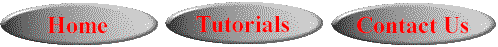©This online SPSS Training Workshop is developed by Dr Carl Lee, Dr Felix Famoye and student assistants Barbara Shelden and Albert Brown , Department of Mathematics, Central Michigan  University. All rights reserved.